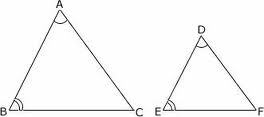# Triangle Similarity

Geometry Level 2THE AREAS OF TWO SIMILAR TRIANGLES ARE IN THE RATIO OF 36:25.

Find the perimeter of the larger triangle, if the perimeter of the smaller is 125.

×